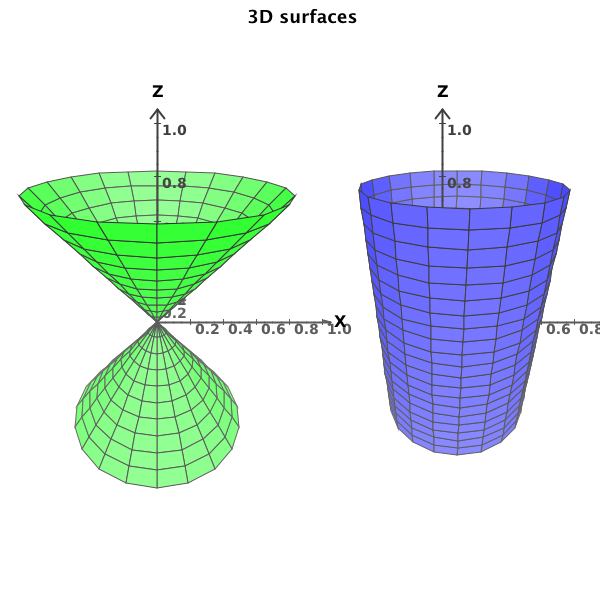# DMelt:Math/2 Parametric Functions

Limitted access. First login to DataMelt if you are a full DataMelt member. Then login to HandWiki as a user.

# Parametric functions

Parametric functions can be constructed using the Java classjhplot.FPR. The variables "u" and "v" are parametric variables used to build "x,y,z". To display such functions, use the canvasjhplot.HPlot3DP.

Lets us show an example of creating two parametric function:

The output of this script is shown hereAs you can see, one can overlay several functions on top of each other and set the transparency level.

Here are some examples: